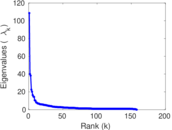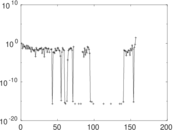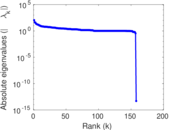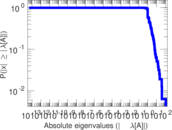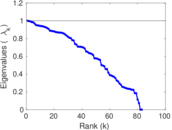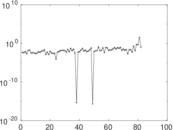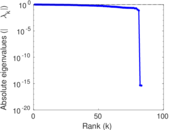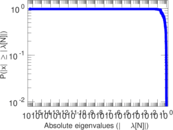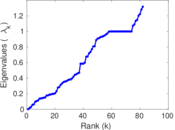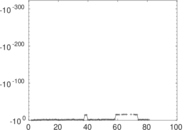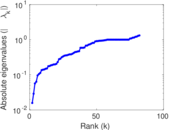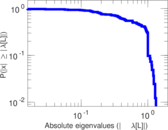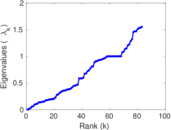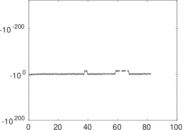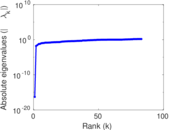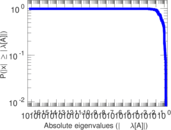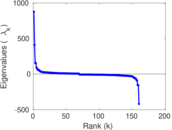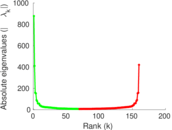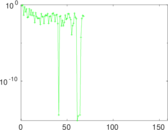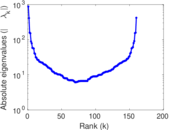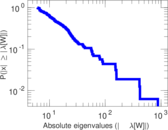# Wiktionary edits (tn)

This is the bipartite edit network of the Tswana Wiktionary. It contains users and pages from the Tswana Wiktionary, connected by edit events. Each edge represents an edit. The dataset includes the timestamp of each edit.

 Code `mtn` Internal name `edit-tnwiktionary` Name Wiktionary edits (tn) Data source http://dumps.wikimedia.org/ AvailabilityDataset is available for download Consistency checkDataset passed all tests Category Authorship network Dataset timestamp 2017-10-20 Node meaning User, article Edge meaning Edit Network formatBipartite, undirected Edge typeUnweighted, multiple edges Temporal dataEdges are annotated with timestamps

## Statistics

 Size n = 882 Left size n1 = 160 Right size n2 = 722 Volume m = 2,716 Unique edge count m̿ = 1,330 Wedge count s = 46,056 Claw count z = 1,879,536 Cross count x = 71,419,969 Square count q = 20,583 4-Tour count T4 = 351,900 Maximum degree dmax = 848 Maximum left degree d1max = 848 Maximum right degree d2max = 46 Average degree d = 6.158 73 Average left degree d1 = 16.975 0 Average right degree d2 = 3.761 77 Fill p = 0.011 513 2 Average edge multiplicity m̃ = 2.042 11 Size of LCC N = 665 Diameter δ = 15 50-Percentile effective diameter δ0.5 = 3.980 52 90-Percentile effective diameter δ0.9 = 7.383 01 Median distance δM = 4 Mean distance δm = 4.880 47 Gini coefficient G = 0.743 058 Balanced inequality ratio P = 0.193 483 Left balanced inequality ratio P1 = 0.142 489 Right balanced inequality ratio P2 = 0.239 691 Relative edge distribution entropy Her = 0.839 079 Power law exponent γ = 3.122 06 Tail power law exponent γt = 2.131 00 Tail power law exponent with p γ3 = 2.131 00 p-value p = 0.162 000 Left tail power law exponent with p γ3,1 = 1.711 00 Left p-value p1 = 0.447 000 Right tail power law exponent with p γ3,2 = 2.311 00 Right p-value p2 = 0.038 000 0 Degree assortativity ρ = −0.146 952 Degree assortativity p-value pρ = 7.308 49 × 10−8 Spectral norm α = 108.595 Algebraic connectivity a = 0.015 751 3 Spectral separation |λ1[A] / λ2[A]| = 2.711 67 Controllability C = 555 Relative controllability Cr = 0.638 665

## Plots

### Fruchterman–Reingold graph drawing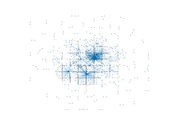### Degree distribution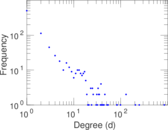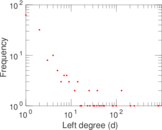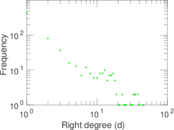### Cumulative degree distribution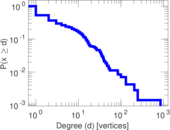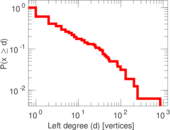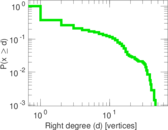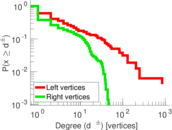### Lorenz curve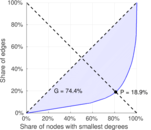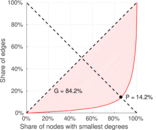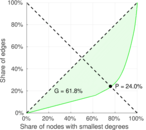### Spectral distribution of the adjacency matrix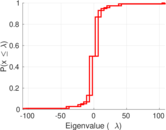### Spectral distribution of the normalized adjacency matrix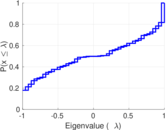### Spectral distribution of the Laplacian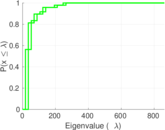### Spectral graph drawing based on the adjacency matrix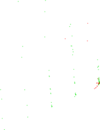### Spectral graph drawing based on the Laplacian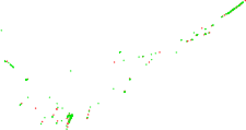### Spectral graph drawing based on the normalized adjacency matrix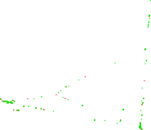### Degree assortativity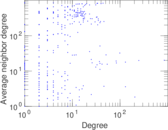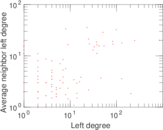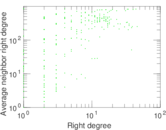### Zipf plot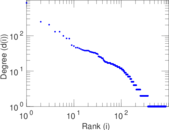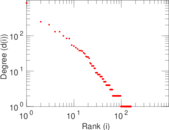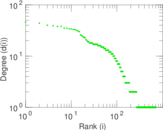### Hop distribution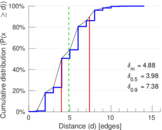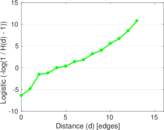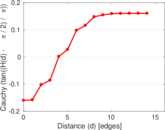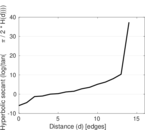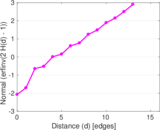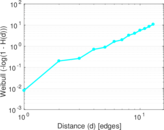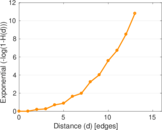### Double Laplacian graph drawing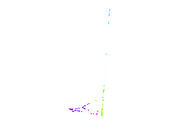### Delaunay graph drawing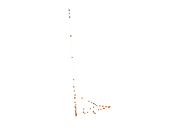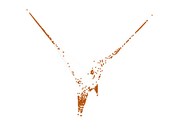### Edge weight/multiplicity distribution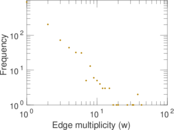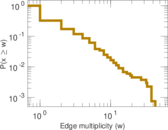### Temporal distribution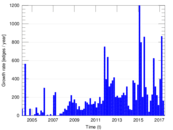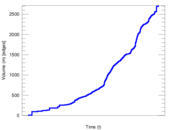### Temporal hop distribution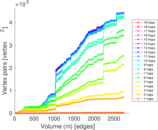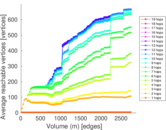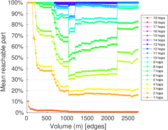### Diameter/density evolution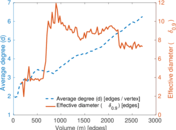### Matrix decompositions plots